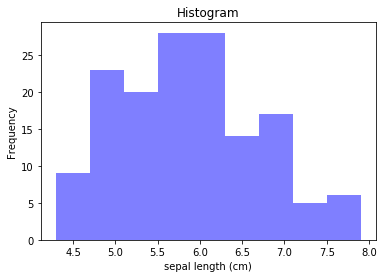# Histogram Chart¶

#### Some preliminaries¶

If you are wondering why this webpage looks the way it does, it might help you to review Anaconda, Jupyter scripts and a basic Python example. You can do so by reviewing the post(s) below.

### Data Exploration with a Histogram¶

We load in a popular predictive modeling dataset called "Iris" using the sklearn library. Then, we utilize a histogram plot to visualize the data and its relationship with the variable that we are trying to predict.

In :
import pandas as pd
data = pd.DataFrame(iris.data, columns=[iris.feature_names]) #loading data into a pandas dataframe (for easier manipulation)

In :
data['y'] = pd.Series(data=iris.target, index=data.index) #the loaded data does not include the target variable for some reason, adding it here
data.describe() #get some basic stats on the dataset

Out:
sepal length (cm) sepal width (cm) petal length (cm) petal width (cm) y
count 150.000000 150.000000 150.000000 150.000000 150.000000
mean 5.843333 3.057333 3.758000 1.199333 1.000000
std 0.828066 0.435866 1.765298 0.762238 0.819232
min 4.300000 2.000000 1.000000 0.100000 0.000000
25% 5.100000 2.800000 1.600000 0.300000 0.000000
50% 5.800000 3.000000 4.350000 1.300000 1.000000
75% 6.400000 3.300000 5.100000 1.800000 2.000000
max 7.900000 4.400000 6.900000 2.500000 2.000000
In :
data.head() #we observe the first few lines of the dataset (always a good idea to get a sense of what is 'in there')

Out:
sepal length (cm) sepal width (cm) petal length (cm) petal width (cm) y
0 5.1 3.5 1.4 0.2 0
1 4.9 3.0 1.4 0.2 0
2 4.7 3.2 1.3 0.2 0
3 4.6 3.1 1.5 0.2 0
4 5.0 3.6 1.4 0.2 0
In :
import matplotlib.pyplot as plt
x = data[["sepal length (cm)"]] #our 'x' axis variable (or whichever column we wish to see the distribution of)

In :
#Now, an issue is that the matplotlib function below uses a Numpy array, and not a dataframe. So we convert the dataframe to array
x_array=x_array = x.values #convert dataframe to array
n, bins, patches = plt.hist(x_array, bins='auto', facecolor='blue', alpha=0.5)
plt.xlabel('sepal length (cm)')
plt.ylabel('Frequency')
plt.title('Histogram');A histogram can be a great tool to quickly observe the distribution of data points.

Helpful tip: you can use the 'snipping tool' in windows to cut/paste any developed charts into your report, presentation, or to save it as a picture and use whereever you like.

### Feedback¶

If you have ideas on how to improve this post, please let me know: https://predictivemodeler.com/feedback/